Next: Higher-order methods Up: Second-order methods Previous: Principal component analysis

### Factor analysis

A method that is closely related to PCA is factor analysis [51,87]. In factor analysis, the following generative model for the data is postulated: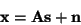(5)

where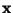is the vector of the observed variables,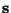is the vector of the latent variables (factors) that cannot be observed,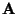is a constant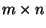matrix, and the vector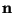is noise, of the same dimension, m, as. All the variables inandare assumed to be Gaussian. In addition, it is usually assumed thathas a lower dimension than. Thus, factor analysis is basically a method of reducing the dimension of the data, in a way similar to PCA.

There are two main methods for estimating the factor analytic model . The first method is the method of principal factors. As the name implies, this is basically a modification of PCA. The idea is here to apply PCA on the datain such a way that the effect of noise is taken into account. In the simplest form, one assumes that the covariance matrix of the noise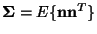is known. Then one finds the factors by performing PCA using the modified covariance matrix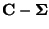, where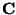is the covariance matrix of. Thus the vectoris simply the vector of the principal components ofwith noise removed. A second popular method, based on maximum likelihood estimation, can also be reduced to finding the principal components of a modified covariance matrix. For the general case where the noise covariance matrix is not known, different methods for estimating it are described in [51,87].

Nevertheless, there is an important difference between factor analysis and PCA, though this difference has little to do with the formal definitions of the methods. Equation (5) does not define the factors uniquely (i.e. they are not identifiable), but only up to a rotation [51,87]. This indeterminacy should be compared with the possibility of choosing an arbitrary basis for the PCA subspace, i.e., the subspace spanned by the first n principal components. Therefore, in factor analysis, it is conventional to search for a 'rotation' of the factors that gives a basis with some interesting properties. The classical criterion is parsimony of representation, which roughly means that the matrixhas few significantly non-zero entries. This principle has given rise to such techniques as the varimax, quartimax, and oblimin rotations . Such a rotation has the benefit of facilitating the interpretation of the results, as the relations between the factors and the observed variables become simpler.Next: Higher-order methods Up: Second-order methods Previous: Principal component analysis
Aapo Hyvarinen
1999-04-23